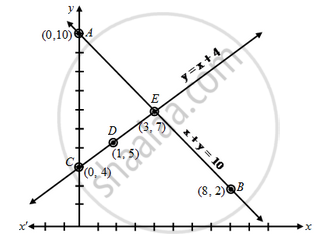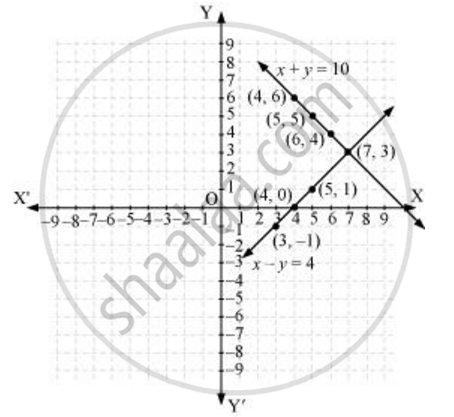# 10 students of class X took part in a Mathematics quiz. If the number of girls is 4 more than the number of boys, find the number of boys and girls who took part in the quiz. - Mathematics

Numerical

10 students of class X took part in a Mathematics quiz. If the number of girls is 4 more than the number of boys, find the number of boys and girls who took part in the quiz.

Form the pair of linear equations in the following problems, and find their solution graphically

10 students of class X took part in a Mathematics quiz. If the number of girls is 4 more than the number of boys, find the number of boys and girls who took part in the quiz.

#### Solution 1

Let the number of boys be x and the number of girls be y.

Then the equations formed are

x + y = 10 ….(1) and y = x + 4 ….(2)

Let us draw the graphs of equations (1) and (2) by finding two solutions for each of the equations.

The solutions of the equations are given.

x + y = 10 ⇒ y = 10 – x

 x 0 8 y 10 2 Points A B

y = x + 4

 x 0 1 3 y 4 5 7 Points C D E

Plotting these points we draw the lines AB and CE passing through them to represent the equations. The two lines AB and Ce intersect at the point E (3, 7). So, x = 3 and y = 7 is the required solution of the pair of linear equations.i.e. Number of boys = 3

Number of girls = 7.

Verification:

Putting x = 3 and y = 7 in (1), we get

L.H.S. = 3 + 7 = 10 = R.H.S., (1) is verified.

Putting x = 3 and y = 7 in (2), we get

7 = 3 + 4 = 7, (2) is verified.

Hence, both the equations are satisfied.

#### Solution 2

Let the number of girls be x and the number of boys be y.

According to the question, the algebraic representation is

x + y = 10

x − y = 4

For x + y = 10,

x = 10 − y

Let the number of girls be x and the number of boys be y.

According to the question, the algebraic representation is

x + y = 10

x − y = 4

For x + y = 10,

x = 10 − y

 x 5 4 6 y 5 6 4

For x − y = 4,

x = 4 + y

 x 5 4 3 y 1 0 −1

Hence, the graphic representation is as follows.From the figure, it can be observed that these lines intersect each other at point (7, 3).

Therefore, the number of girls and boys in the class are 7 and 3 respectively.

Concept: Graphical Method of Solution of a Pair of Linear Equations
Is there an error in this question or solution?

#### APPEARS IN

NCERT Class 10 Maths
Chapter 3 Pair of Linear Equations in Two Variables
Exercise 3.2 | Q 1.1 | Page 49
RD Sharma Class 10 Maths
Chapter 3 Pair of Linear Equations in Two Variables
Exercise 3.2 | Q 34.1 | Page 31